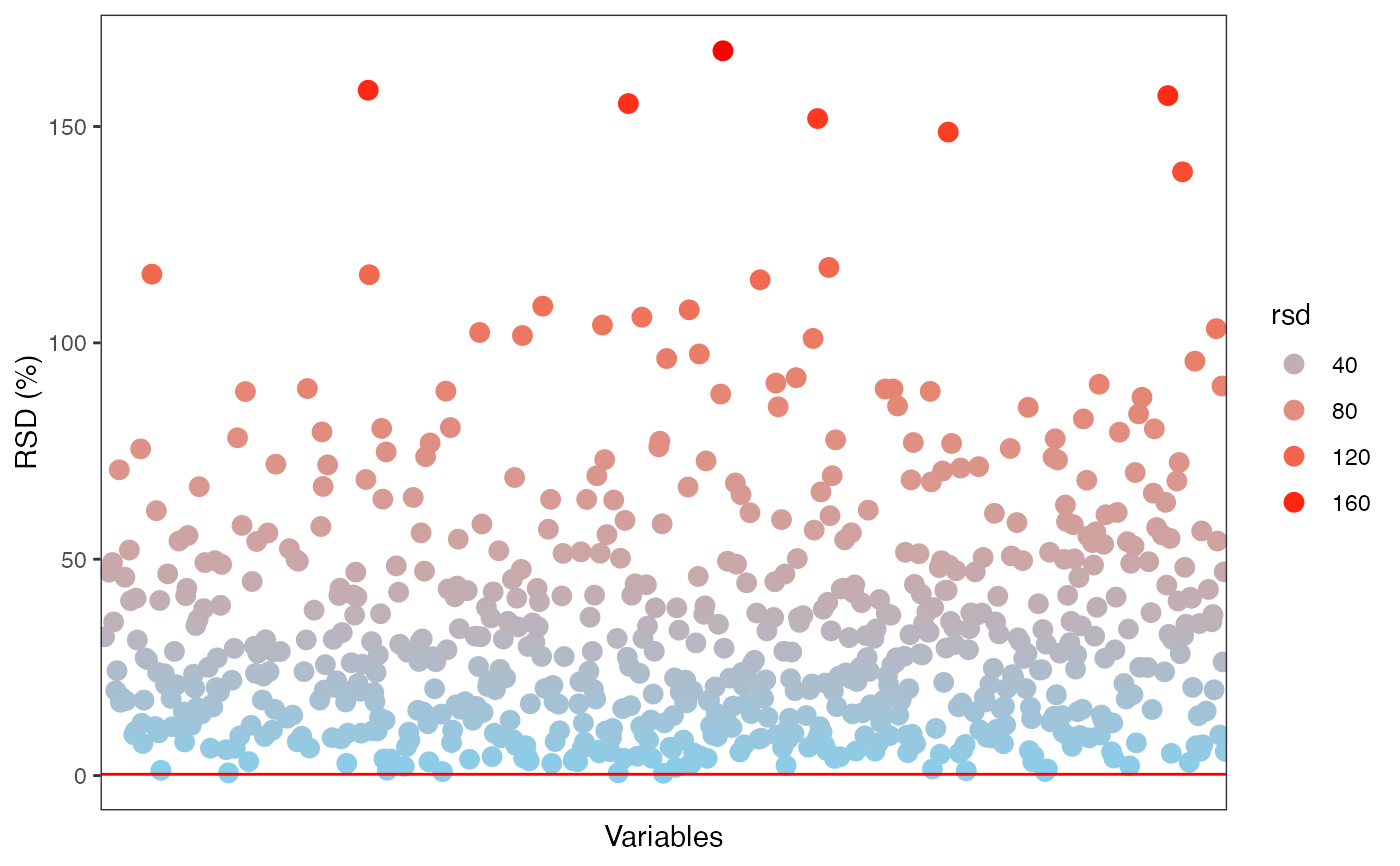RSD plot for each variable.

massqc_rsd_plot(
object,
color_by,
order_by = "variable_id",
show_x_text = FALSE,
show_x_ticks = FALSE,
desc = FALSE,
point_alpha = 0.8,
point_size = 3
)

## Arguments

object

tidymass-class object.

color_by

which column (sample_info) is used to color samples

order_by

which column (sample_info) is used to order samples

show_x_text

show_x_text

show_x_ticks

show_x_ticks

desc

desc

point_alpha

point_alpha

point_size

point_size

ggplot2 plot.

## Author

Xiaotao Shen shenxt1990@163.com

## Examples

library(massdataset)
library(ggplot2)
data("expression_data")
data("sample_info")
data("variable_info")

object =
create_mass_dataset(
expression_data = expression_data,
sample_info = sample_info,
variable_info = variable_info
)

object %>%
massqc_rsd_plot()
#> Warning: Removed 140 rows containing missing values (geom_point).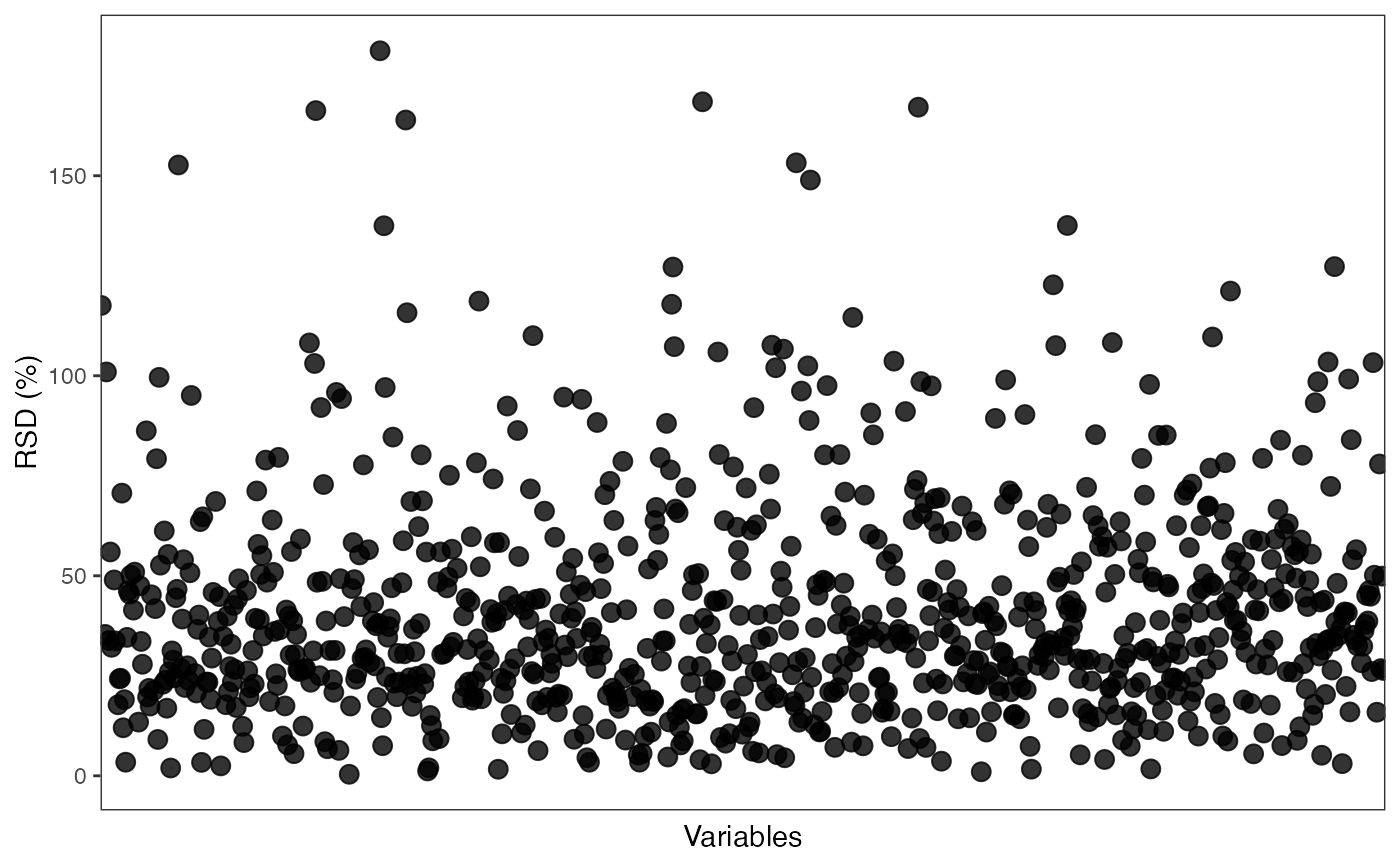object %>%
massqc_rsd_plot(color_by = "rsd")
#> Warning: Removed 140 rows containing missing values (geom_point).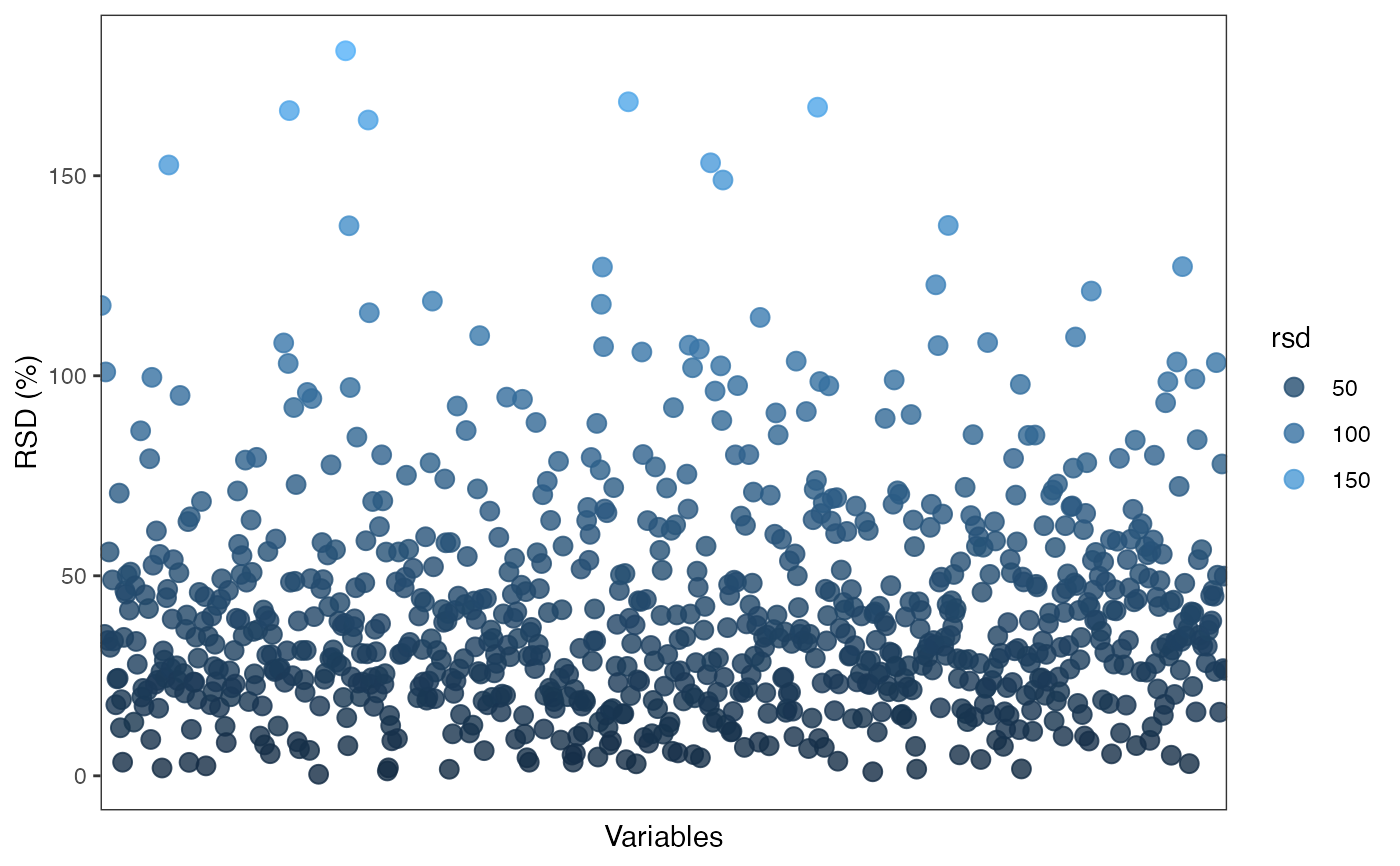object %>%
massqc_rsd_plot(color_by = "rsd", order_by = "rsd")
#> Warning: Removed 140 rows containing missing values (geom_point).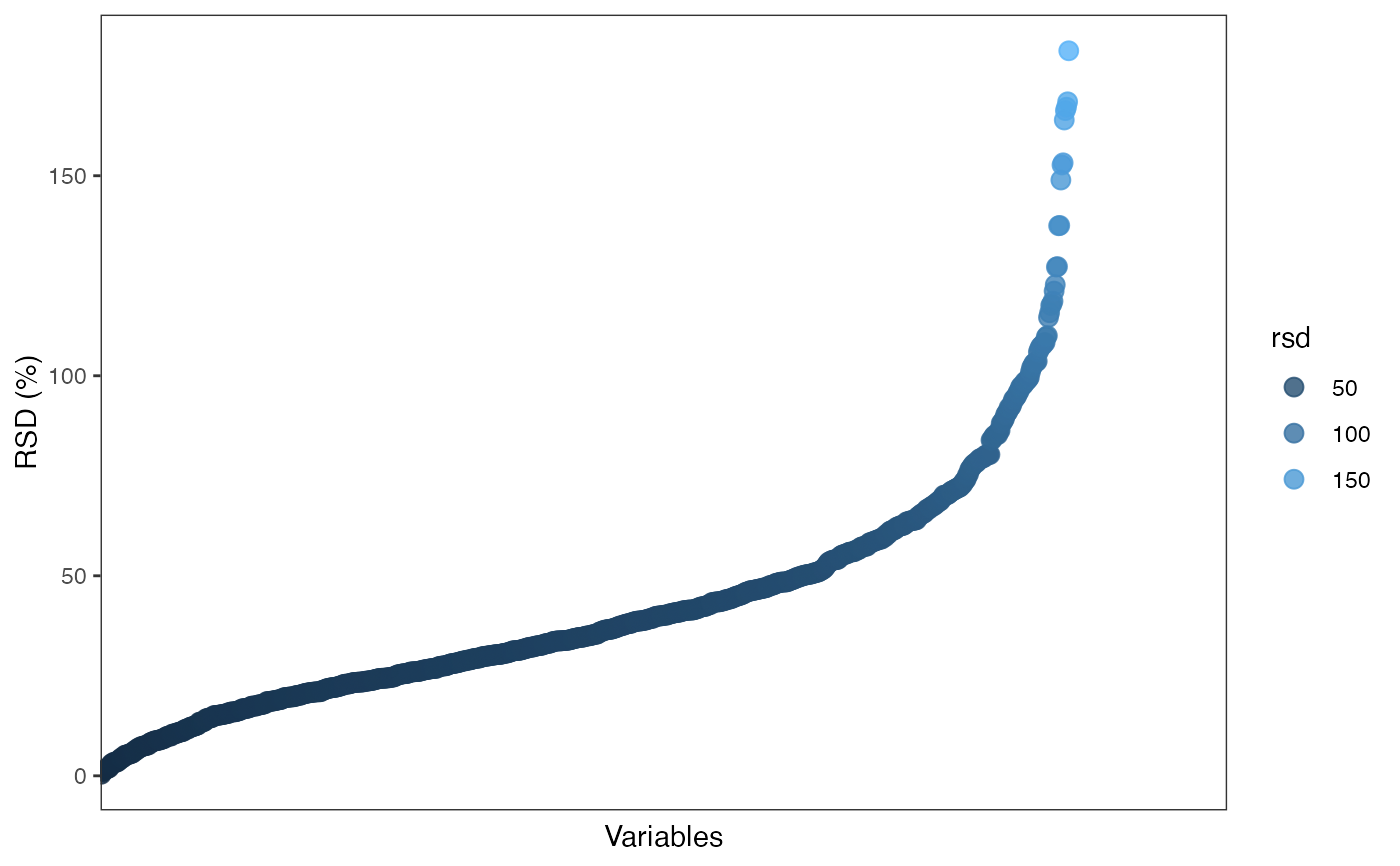object %>%
massqc_rsd_plot(color_by = "rsd", point_alpha = 1) +
scale_color_gradient(low = "skyblue", high = "red") +
geom_hline(yintercept = 0.3, color = "red")
#> Warning: Removed 140 rows containing missing values (geom_point).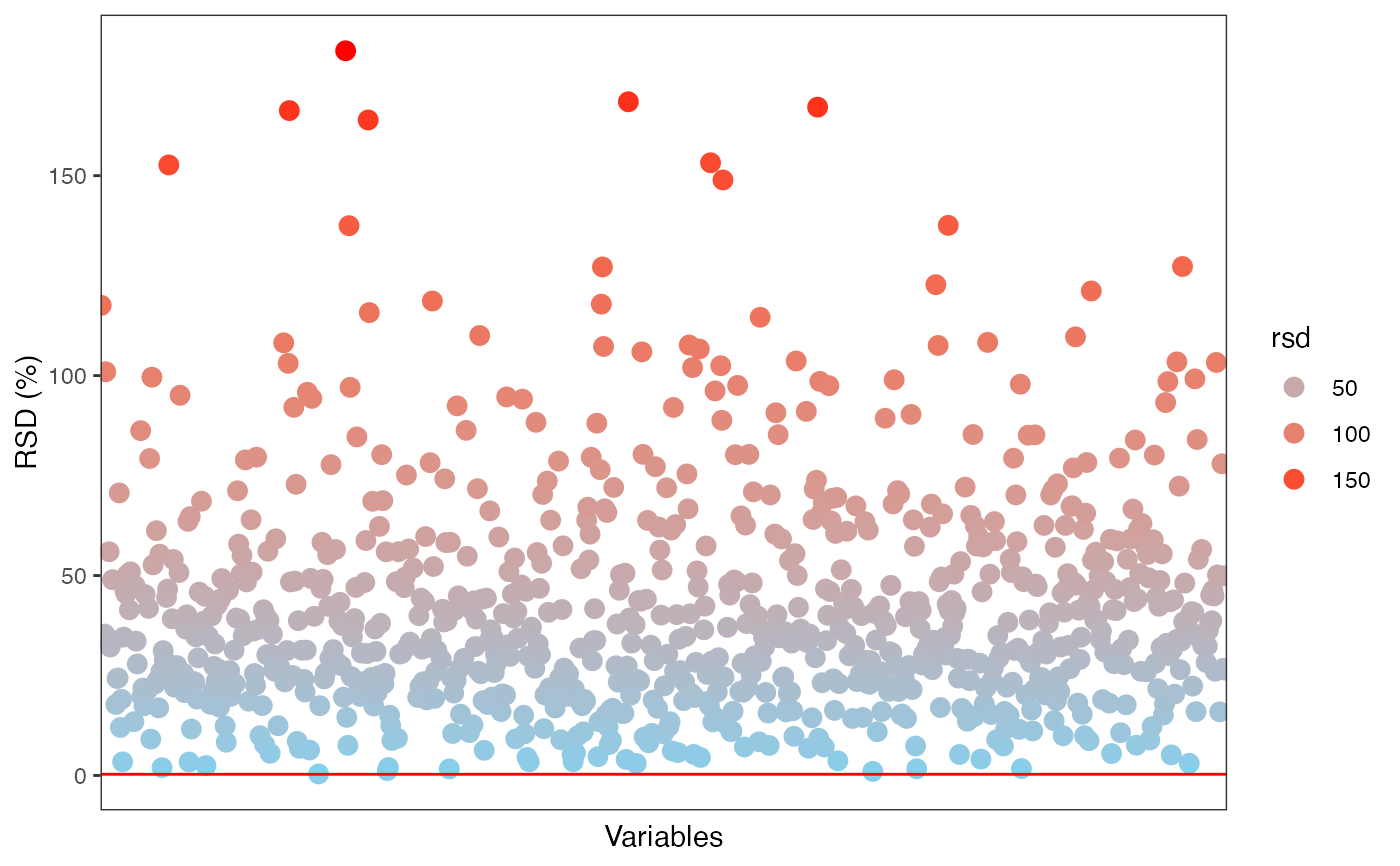object %>%
activate_mass_dataset(what = "sample_info") %>%
filter(class == "Subject") %>%
massqc_rsd_plot(color_by = "rsd", point_alpha = 1) +
scale_color_gradient(low = "skyblue", high = "red") +
geom_hline(yintercept = 0.3, color = "red")
#> Warning: Removed 325 rows containing missing values (geom_point).